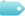# 惠斯通电桥的工作原理、电路图及电阻测试方法

367 2022-06-20 来源: IC先生 作者: IC先生### 惠斯通电桥电路布置### 惠斯通电桥推导公式

I1=V/P+Q、I2=V/R+S

I1Q= VQ/P+Q…………………………..(1)

D点相对于C的电位是电阻S两端的电压降，则等式为：

I2S=VS/R+S…………………………..(2)

VQ/P+Q = VS/R+S

Q/P+Q = S/R+S

P+Q/Q=R+S/S

P/Q+1=R/S+1

P/Q=R/S

R=SxP/Q

### 惠斯通电桥示例演示Vc= (R2/(R1+R2)) X Vs，其中：

R2=120ohms, R1=80 ohms, Vs=100

Vc= (120/(80+ 120)) X 100 = 60伏

VD = R4/(R3+R4) X Vs，代入值得到：

VD= 160/ (480+160) X 10 =25伏特

Vout= VC-VD=60特 - 25 = 35

R4= R2 R3/R1 = 120X480 / 80 = 720 ohms。

### 惠斯通电桥的应用LDR是一种无源电阻传感器，用于将可见光水平转换为电阻变化和电压变化。LDR可用于测量和监控光强度水平。LDR在100勒克斯的光强度下具有大约900Ω的暗光或暗光下的几兆欧电阻，而在强光下低至约 30欧姆。通过在惠斯通电桥电路中连接光敏电阻，可以测量和监测光照水平的变化。

### 总结